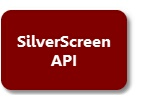﻿ mark_area
SilverScreen Solid Modeler

# mark_area# mark_areaint mark_area ( char *message, double *x1, double *y1, double *x2, double *y2 )

char   *message;  // prompt message

double *x1;       // address of normalized left coordinate

double *y1;       // address of normalized upper coordinate

double *x2;       // address of normalized right coordinate

double *y2;       // address of normalized lower coordinate

Synopsis

#include "silver.h"

The mark_area function displays message , and then allows the user to select a rectangular area from the current screen. The coordinates pairs (x1 ,y1) and (x2 ,y2) specify the corners of the rectangular area as measured in normalized coordinates relative to the current screen; that is (0,0) is the lower left corner of the screen, (0,1) is the upper left corner, (1,0) is the lower right corner and (1,1) is the upper right corner of the screen.

Parameters

message is a null-terminated string containing the prompt message. x1 , x2 , y1 and x2 are all addresses of doubles that are to receive the normalized area coordinates.

Return Value

If the selection is successfully made, the coordinates of the area are stored into the doubles at x1 , y1 , x2 and y2 , and mark_area returns 1; otherwise, mark_area returns 0.

Example

The following example uses mark_area to zoom the current window:

 C / C++ Code double d1, d2, d3, d4;    if ( mark_area ( "Mark area to zoom", &d1, &d2, &d3, &d4 ) )     ss_command ( "zoom area %f,%f %f,%f", d1, d2, d3, d4 );